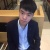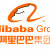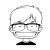0+0+0+0+0+0+0+0+0+0+0+0+0+0+0+0+0+0+【每秒7亿次请求，阿里新一代数据库如何支撑？ | 详情传送门：http://t.cn/AiDq3BqQ】 Lindorm，就是云操作系统飞天中面向大数据存储处理的重要组成部分。Lindorm是基于HBase研发的、面向大数据领域的分布式NoSQL数据库，集大规模、高吞吐、快速灵活、实时混合能力于一身，面向海量数据场景提供世界领...全文0+0+0+0+0+0+0+0+0+0+0+0+0+0+0+0+0+0+0+0+0+0+0+0+0+0+0+
Top